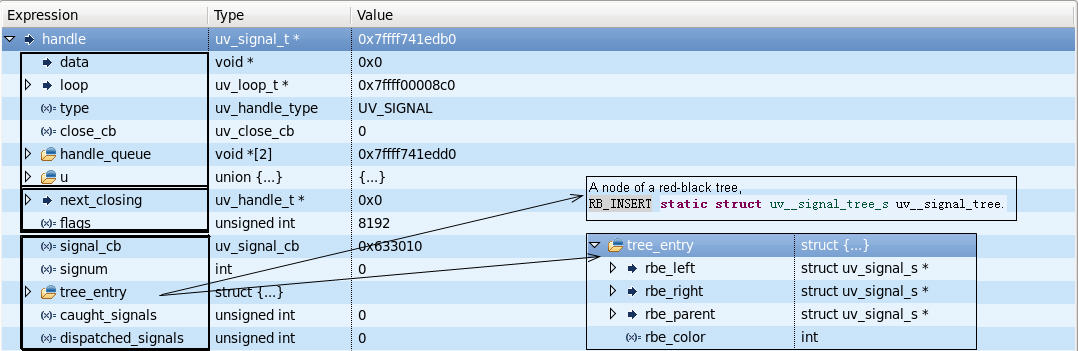# The target of the design:

• Asynchronously call signal processing function.
• In the loop thread call signal processing function.

# struct uv_signal_t``````typedef struct {
uv_signal_t* handle;
int signum;
} uv__signal_msg_t;

struct uv_loop_s {
... ...
/* a pipe, signal_pipefd refers to the read end of the pipe,
* signal_pipefd refers to the write end of the pipe.
* uv__io_poll watches the read end, while signal action uses the
* write end. When catching a signal, write a uv__signal_msg_t to
* the write end.
*/
int signal_pipefd;
uv__io_t signal_io_watcher;
... ...
};
``````

# Interface

``````UV_EXTERN int uv_signal_init(uv_loop_t* loop, uv_signal_t* handle);
UV_EXTERN int uv_signal_start(uv_signal_t* handle,
uv_signal_cb signal_cb,
int signum);
UV_EXTERN int uv_signal_stop(uv_signal_t* handle);

/* Use kill(), killpg() or raise() to actively raise a signal. */
``````

# Flow

## 1. set the action for signal signum

``````static void uv__signal_handler(int signum) {
uv__signal_msg_t msg;
uv_signal_t* handle;
int saved_errno;

saved_errno = errno;
memset(&msg, 0, sizeof msg);

if (uv__signal_lock()) {
errno = saved_errno;
return;
}

/* Traversing the red-black tree. */
for (handle = uv__signal_first_handle(signum);
handle != NULL && handle->signum == signum;
handle = RB_NEXT(uv__signal_tree_s, &uv__signal_tree, handle)) {
int r;

msg.signum = signum;
msg.handle = handle;

/* write() should be atomic for small data chunks, so the entire message
* should be written at once. In theory the pipe could become full, in
* which case the user is out of luck.
*/
do {
r = write(handle->loop->signal_pipefd, &msg, sizeof msg);
} while (r == -1 && errno == EINTR);

assert(r == sizeof msg ||
(r == -1 && (errno == EAGAIN || errno == EWOULDBLOCK)));

if (r != -1)
handle->caught_signals++;
}

uv__signal_unlock();
errno = saved_errno;
}

static int uv__signal_register_handler(int signum) {
/* When this function is called, the signal lock must be held. */
struct sigaction sa;

/* XXX use a separate signal stack? */
memset(&sa, 0, sizeof(sa));
abort();
sa.sa_handler = uv__signal_handler;

/* XXX save old action so we can restore it later on? */
if (sigaction(signum, &sa, NULL))
return -errno;

return 0;
}
``````

## 2. set the callback for signal I/O fd

``````uv__io_init(&loop->signal_io_watcher, uv__signal_event, loop->signal_pipefd);
uv__io_start(loop, &loop->signal_io_watcher, UV__POLLIN);
``````

## 3. call the callback of signal I/O fd

``````static void uv__signal_event(uv_loop_t* loop,
uv__io_t* w,
unsigned int events) {
uv__signal_msg_t* msg;
uv_signal_t* handle;
char buf[sizeof(uv__signal_msg_t) * 32];
size_t bytes, end, i;
int r;

bytes = 0;
end = 0;

do {
r = read(loop->signal_pipefd, buf + bytes, sizeof(buf) - bytes);

if (r == -1 && errno == EINTR)
continue;

if (r == -1 && (errno == EAGAIN || errno == EWOULDBLOCK)) {
/* If there are bytes in the buffer already (which really is extremely
* unlikely if possible at all) we can't exit the function here. We'll
* spin until more bytes are read instead.
*/
if (bytes > 0)
continue;

/* Otherwise, there was nothing there. */
return;
}

/* Other errors really should never happen. */
if (r == -1)
abort();

bytes += r;

/* `end` is rounded down to a multiple of sizeof(uv__signal_msg_t). */
end = (bytes / sizeof(uv__signal_msg_t)) * sizeof(uv__signal_msg_t);

for (i = 0; i < end; i += sizeof(uv__signal_msg_t)) {
msg = (uv__signal_msg_t*) (buf + i);
handle = msg->handle;

if (msg->signum == handle->signum) {
assert(!(handle->flags & UV_CLOSING));
handle->signal_cb(handle, handle->signum);
}

handle->dispatched_signals++;

/* If uv_close was called while there were caught signals that were not
* yet dispatched, the uv__finish_close was deferred. Make close pending
* now if this has happened.
*/
if ((handle->flags & UV_CLOSING) &&
(handle->caught_signals == handle->dispatched_signals)) {
uv__make_close_pending((uv_handle_t*) handle);
}
}

bytes -= end;

/* If there are any "partial" messages left, move them to the start of the
* the buffer, and spin. This should not happen.
*/
if (bytes) {
memmove(buf, buf + end, bytes);
continue;
}
} while (end == sizeof buf);
}
``````

# Reference

``````/* Send signal SIG to process number PID.  If PID is zero,
send SIG to all processes in the current process's process group.
If PID is < -1, send SIG to all processes in process group - PID.  */
extern int kill (__pid_t __pid, int __sig) __THROW;

/* Send SIG to all processes in process group PGRP.
If PGRP is zero, send SIG to all processes in
the current process's process group.  */
extern int killpg (__pid_t __pgrp, int __sig) __THROW;

/* Raise signal SIG, i.e., send SIG to yourself.  */
extern int raise (int __sig) __THROW;

/* Set all signals in SET.  */
extern int sigfillset (sigset_t *__set) __THROW __nonnull ((1));

/* Modify the signal mask for the calling thread.  The arguments have
the same meaning as for sigprocmask(2). */